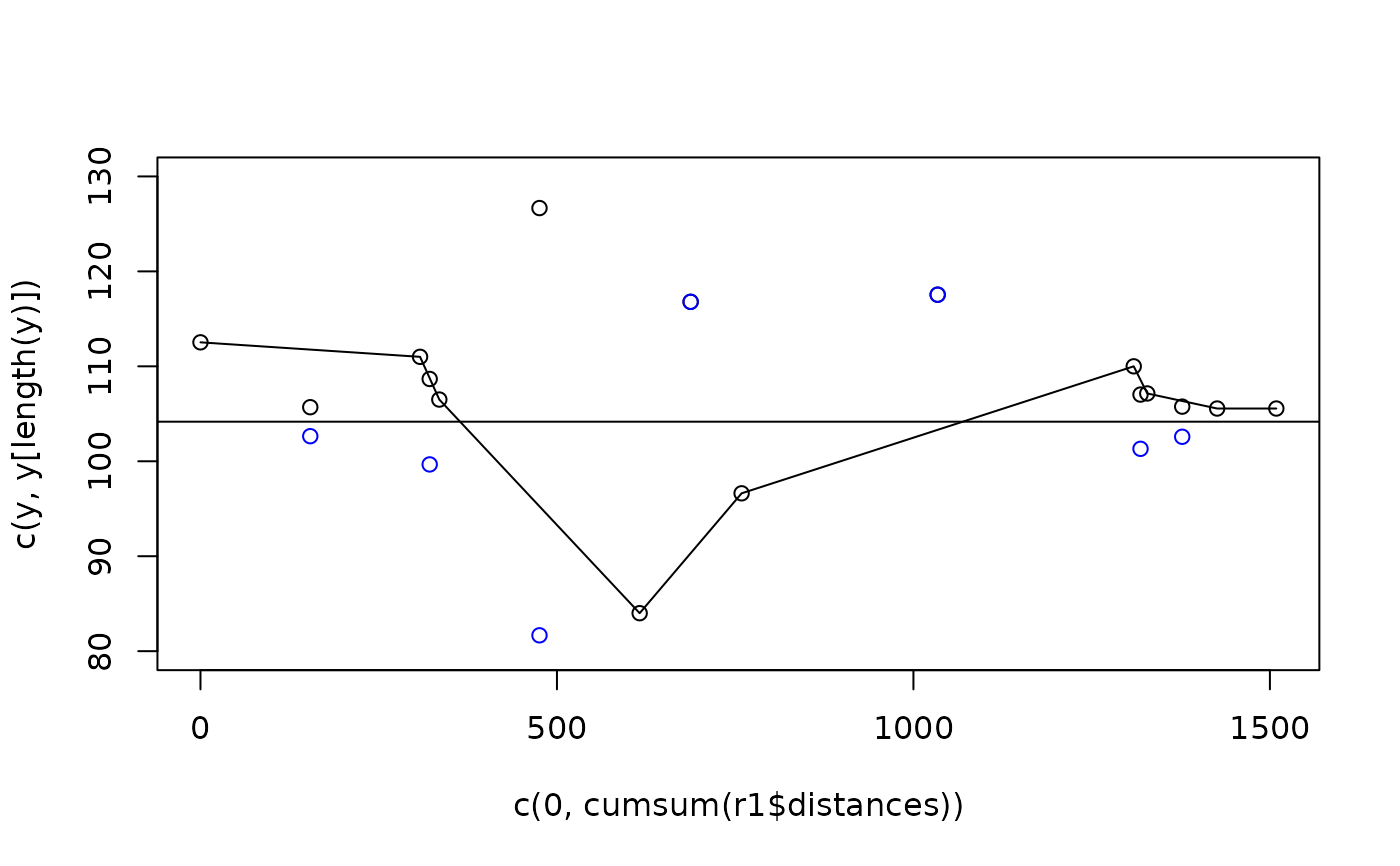This function calculates a simple rolling mean in base R. It is useful for calculating route characteristics such as mean distances of segments and changes in gradient.

## Usage

``route_rolling_diff(x, lag = 1, abs = TRUE)``

## Arguments

x

Numeric vector to smooth

lag

The window size of the smoothing function. The default, 3, will take the mean of values before, after and including each value.

abs

Should the absolute (always positive) change be returned? True by default

Other route_funs: `route_average_gradient()`, `route_rolling_average()`, `route_rolling_gradient()`, `route_sequential_dist()`, `route_slope_matrix()`, `route_slope_vector()`

## Examples

``````r1 <- od_data_routes[od_data_routes\$route_number == 2, ]
y <- r1\$elevations
route_rolling_diff(y, lag = 1)
#>   1.526316  4.500000 22.500000 12.625000 13.375000  2.857143  1.587302
#>         NA
route_rolling_diff(y, lag = 2)
#>   6.026316 27.000000  9.875000 26.000000 10.517857  4.444444        NA
#>         NA
r1\$elevations_diff_1 <- route_rolling_diff(y, lag = 1)
r1\$elevations_diff_n <- route_rolling_diff(y, lag = 1, abs = FALSE)
d <- cumsum(r1\$distances) - r1\$distances / 2
diff_above_mean <- r1\$elevations_diff_1 + mean(y)
diff_above_mean_n <- r1\$elevations_diff_n + mean(y)
plot(c(0, cumsum(r1\$distances)), c(y, y[length(y)]), ylim = c(80, 130))
lines(c(0, cumsum(r1\$distances)), c(y, y[length(y)]))
points(d, diff_above_mean)
points(d, diff_above_mean_n, col = "blue")
abline(h = mean(y))``````